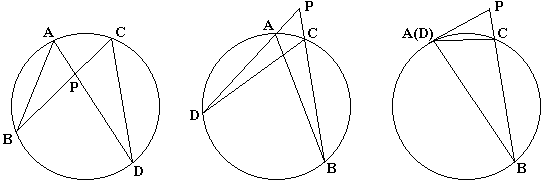# Power of a Point TheoremGiven a point $P$ and a circle, pass two lines through $P$ that intersect the circle in points $A$ and $D$ and, respectively, $B$ and $C.$ Then $AP\cdot DP = BP\cdot CP.$

The point $P$ may lie either inside or outside the circle. The line through $A$ and $D$ (or that through $B$ and $C$ or both) may be tangent to the circle, in which case $A$ and $D$ coalesce into a single point. In all the cases, the theorem holds and is known as the Power of a Point Theorem.

When the point $P$ is inside the circle, the theorem is also known as the Theorem of Intersecting Chords (or the Intersecting Chords Theorem) and has a beautiful interpretation. When the point $P$ is outside the circle, the theorem becomes the Theorem of Intersecting Secants (or the Intersecting Secants Theorem.)

The proof is exactly the same in all three cases mentioned above. Since triangles ABP and CDP are similar, the following equality holds:

$\displaystyle\frac{AP}{CP} = \frac{BP}{DP},$

which is equivalent to the statement of the theorem: $AP\cdot DP = BP\cdot CP.$

The common value of the products then depends only on $P$ and the circle and is known as the Power of Point $P$ with respect to the (given) circle. Note that, when $P$ lies outside the circle, its power equals the length of the square of the tangent from P to the circle. For example, if $B=C$ so that $BP$ is tangent to the circle $AP\cdot DP=BP^{2}.$

Sometimes it is useful to employ signed segments. The convenience is that it is possible to tell points inside the circle from the points outside the circle. The power of a point inside the circle is negative, whereas that of a point outside the circle is positive. This is exactly what one obtains from the algebraic definition of the power of a point.

The theorem is reversible: Assume points $A,$ $B,$ $C,$ and $D$ are not collinear. Let $P$ be the intersection of $AD$ and $BC$ such that $AP\cdot DP = BP\cdot CP.$ Then the four points $A,$ $B,$ $C,$ and $D$ are concyclic. To see that draw a circle through, say, $A,$ $B,$ and $C.$ Assume it intersects $AP$ at $D'.$ Then, as was shown above, $AP\cdot D'P = BP\cdot CP,$ from which $D = D'.$ (If, say, $B$ and $C$ coincide, draw the circle through $A$ tangent to $PB$ at $B.)$### Power of a Point wrt a Circle